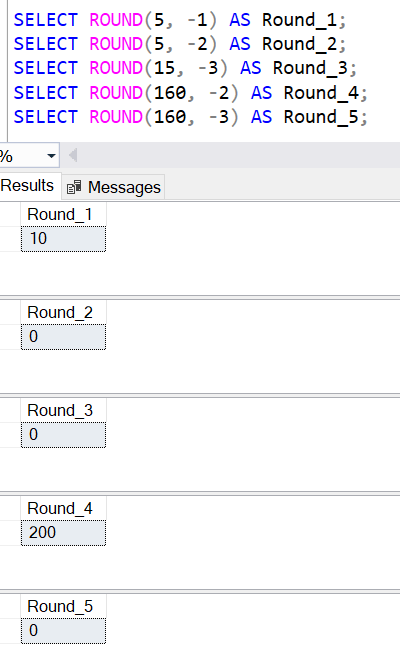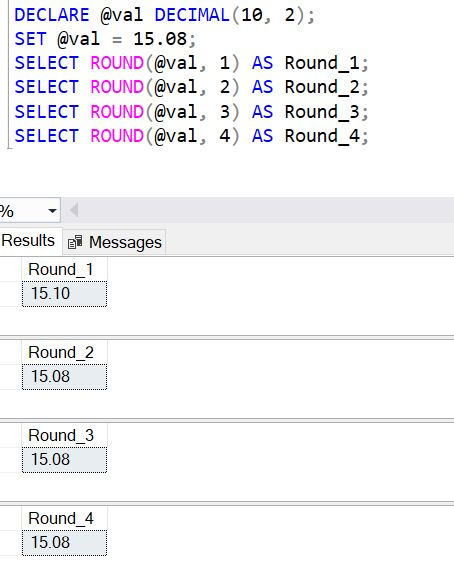Home » SQL ROUND Function

# SQL ROUND Function### The SQL ROUND function is a SQL Mathematical Function which is used to round a number to a specified length or precision.

It returns a numeric value.

It always returns a value. It returns 0, If length is negative and larger than the number of digits before the decimal point.

```SYNTAX
ROUND ( numeric_expression , length [ ,function ] )```

numeric_expression: It can be a number or numeric data type expression. It can not be a Bit data type.

Length: It is the number of decimal places to which we want to round the numeric_expression. Length number can be positive and negative of type tinyint, smallint, or int.

• If value for length parameter is a positive number, then Round function will round the numeric_expression to the number of the decimal point specified as length.
• If value for length parameter is a positive number, Round function will round the numeric_expression to the left side of the decimal point.

Function: It is an optional parameter and specifies the truncation point of the value. The default value for this parameter is zero. If value is not specifed to this parameter then it rounds the numeric_expression. If any value other than 0 is specified to the function, then it truncates the numeric_expression.

Lets look at an example of using SQL Server Round Function.

#### Using Round function with positive Inetger and positive length

For positive integer with positive length, Round function always returns the same number without any rounding.

SELECT ROUND(5, 0) AS Round_0;
SELECT ROUND(5, 1) AS Round_1;
SELECT ROUND(5, 2) AS Round_2;
SELECT ROUND(15, 3) AS Round_3;
SELECT ROUND(155, 4) AS Round_4;

As you can see, round function returns same number without rounding.#### Using Round function with positive Integer and negative length

For positive integer with Negative length, Rounds function rounds the number to the nearest tens place, and when length is larger than the number of digits before the decimal point, then Round function always returns 0.

```SELECT ROUND(5, -1) AS Round_1;
SELECT ROUND(5, -2) AS Round_2;
SELECT ROUND(15, -3) AS Round_3;
SELECT ROUND(160, -2) AS Round_4;
SELECT ROUND(160, -3) AS Round_5;```

As you can see output of Round Function:

For SELECT ROUND(5, -1), positive integer value 5 and negative length -2, round function rounds up the value to 10.

For SELECT ROUND(160, -3), positive integer value 160 and negative length -2, round function rounds up the value to 200.#### Using Round function with decimal value and positive length

Following  T-SQL uses round function and round up to the nearest values for specified length number.

```DECLARE @val DECIMAL(10, 2);
SET @val = 15.08;
SELECT ROUND(@val, 1) AS Round_1;
SELECT ROUND(@val, 2) AS Round_2;
SELECT ROUND(@val, 3) AS Round_3;
SELECT ROUND(@val, 4) AS Round_4;```

As you can see that with decimal values round up to the nearest value as per the given length.